Function Repository Resource:

# DiscreteHausdorffDistance

Find the Hausdorff distance between two sets of elements

Contributed by: Sjoerd Smit
 ResourceFunction["DiscreteHausdorffDistance"][set1, set2] returns the Hausdorff distance between two sets, which is the maximum distance you need to travel from a point in one set to reach a point in the other.

## Details and Options

Given a distance function d that measures the minimum distance from an element to a set, the Hausdorff distance is given by:ResourceFunction["DiscreteHausdorffDistance"] takes the same options as Nearest.

## Examples

### Basic Examples (2)

Measure the distance between two sets of numbers:

 In:=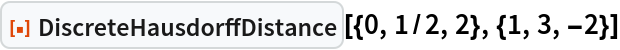Out=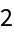This is the maximum of the following distances:

 In:=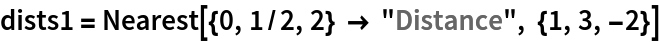Out=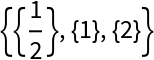In:=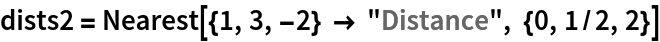Out=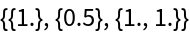In:=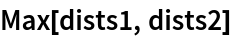Out=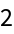### Scope (1)

Use a custom DistanceFunction for sets of strings:

 In:=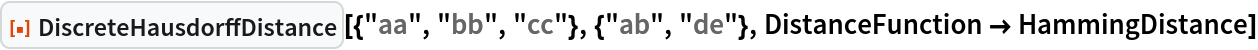Out=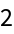### Applications (2)

Compute the distance between sets of points in 2D space:

 In:=Out=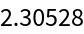Show the maximum distances from one set to the other as arrows. DiscreteHausdorffDistance is equal to the longest of these two arrows:

 In:=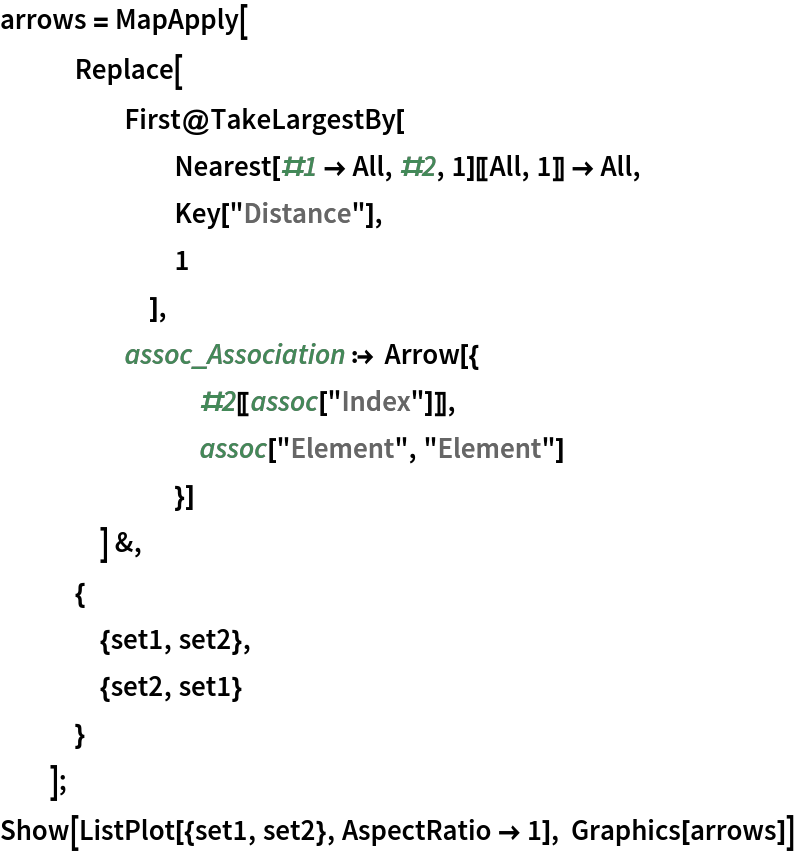Out=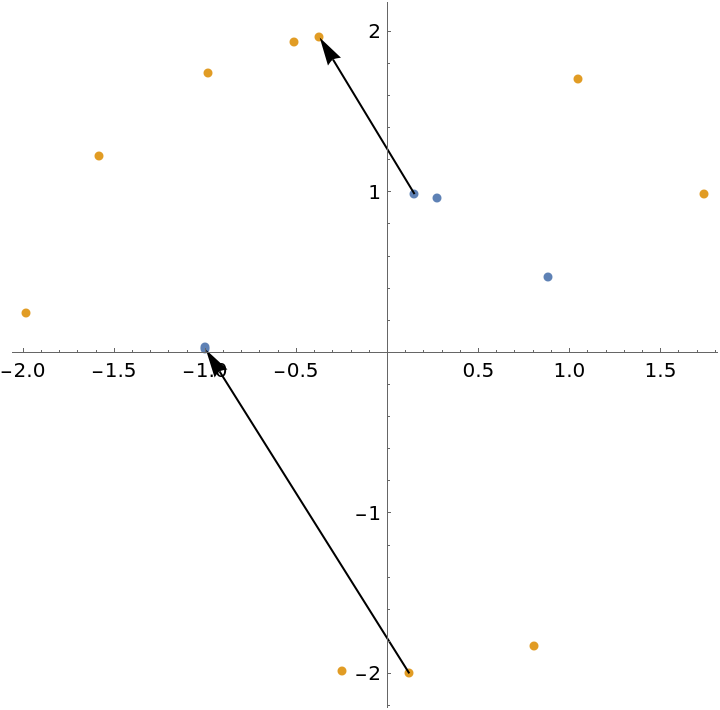Sjoerd Smit

## Version History

• 1.0.0 – 26 April 2023